# Case Study¶

We use a simple example to demonstrate how to work with BleemCube API and Crystallon tools to create the lattice.

Assuming in a product design task, we would like to create an Inconel 718 metal cube filled with lattice structure, and the cube should produce an overall effective elastic modulus 30.13GPa (example only, no special meaning or implication). The question is what the unit cell size and beam thickness are. Design is about iterative process. We could either keep testing the prototypes of the blocks (manufactured through 3D printer) till the desired solution is found; or we could repeat such test virtually, say FEA modelling technique. Both methods will cost many time and efforts that development works would like to avoid. Here we propose a new way of designing the lattice structure, and user should have the initial design in a few seconds.

## Lattice Cube¶

We built a simple cube model using Grasshopper. The screen shot below showed the base for the cube geometry. This base enables user to adjust the cube size (e.g. height, width and depth) and the position.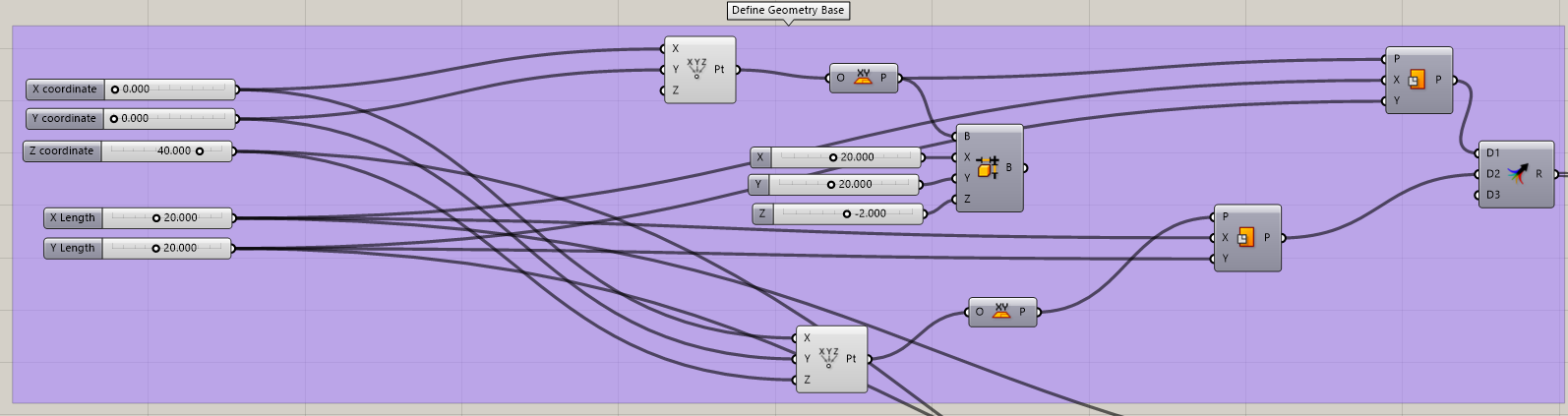The generated top and bottom surfaces are as shown below.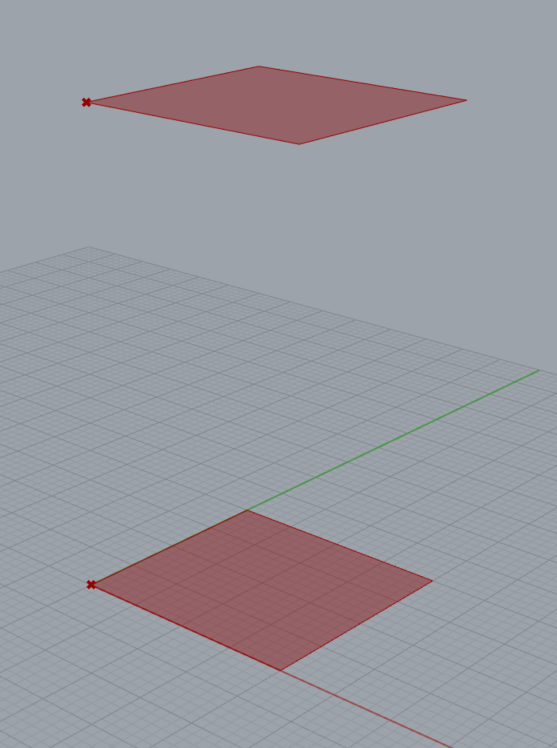We then use Crystallon to vowelize the space between the top and bottom surfaces, and fill the selected unit lattice. Here we picked the Prim. Cubic.In order to define the unit cell geometric features, we apply the tool “TargetE Constraint” to search entire design domain.And in a few seconds, we should have the following results. Please note that Cube use SI units, we convert m to mm. User also should check the results `E_diff` which shows the absolute difference between the target number and solution. The smaller `E_diff` means the better results. In this case, `E_diff` is about `65`.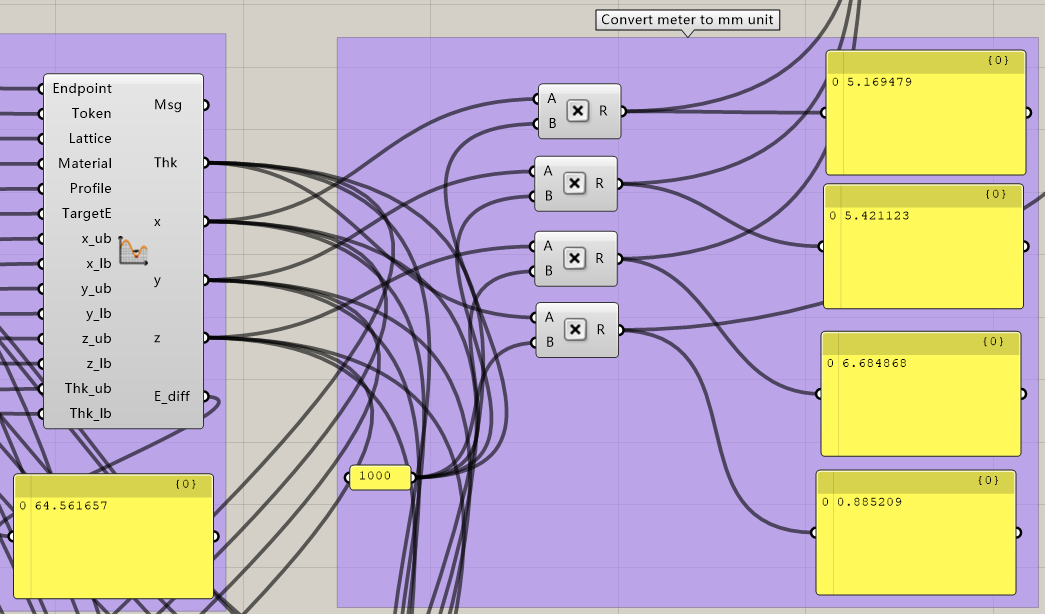Once we feed the data into the Crystallon section, we should be able to see the following geometry.Adding the pipe with calculated thickness (i.e. the radius for the profile “C”), we should see the cube below. In other words, the lattice cube below produces an overall effective elastic modulus 30.13GPa with the base material Inconel 718.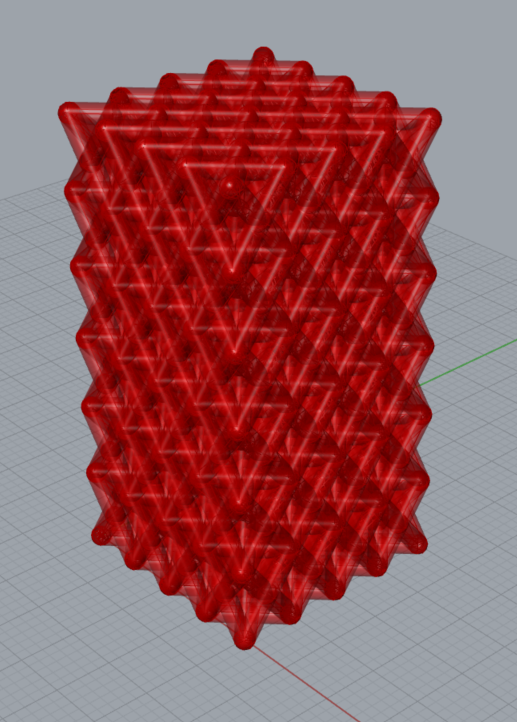We can check the calculated `E` through Cube API. As demonstrated below, the elastic modulus is 30.13GPa.## Verification¶

We could verify the results by using FEA software. We solve the compression on the lattice using a popular commercial solver (i.e. solver A) and a popular opensource solver (i.e. solver B). One needs note that, above geometry used the round number on the actual number of cells in each direction. Therefore, for the better results, the geometric model for solver A is reconstructed 5 units cells in X direction, 5 unit cells in Y direction, and 5 unit cells in Z direction. For solver B, the number of unit cell in Z direction is 10. The material data can be extracted using the tool “Material Data”. The comparison of the engineering stress-strain curves is shown below. The Cube API calculated curve lays closing to the solver B results, and has some differences to the solver A results, but generally follows the trends of the curve. The Cube API calculation fits to the FEA results.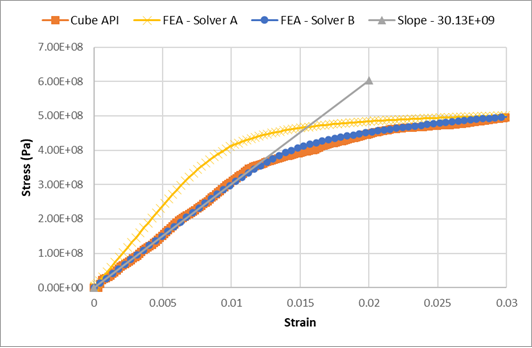Please note that the modelling software has its own algorithm to solve specific problem. It often recommend to tune the system parameters (e.g. material parameters) to fits the best of correlation. For improved the reliability and accuracy of the cube API’s calculation, we highly recommend the customized Cube API that calibrates the API calculation with the actual material characteristics.